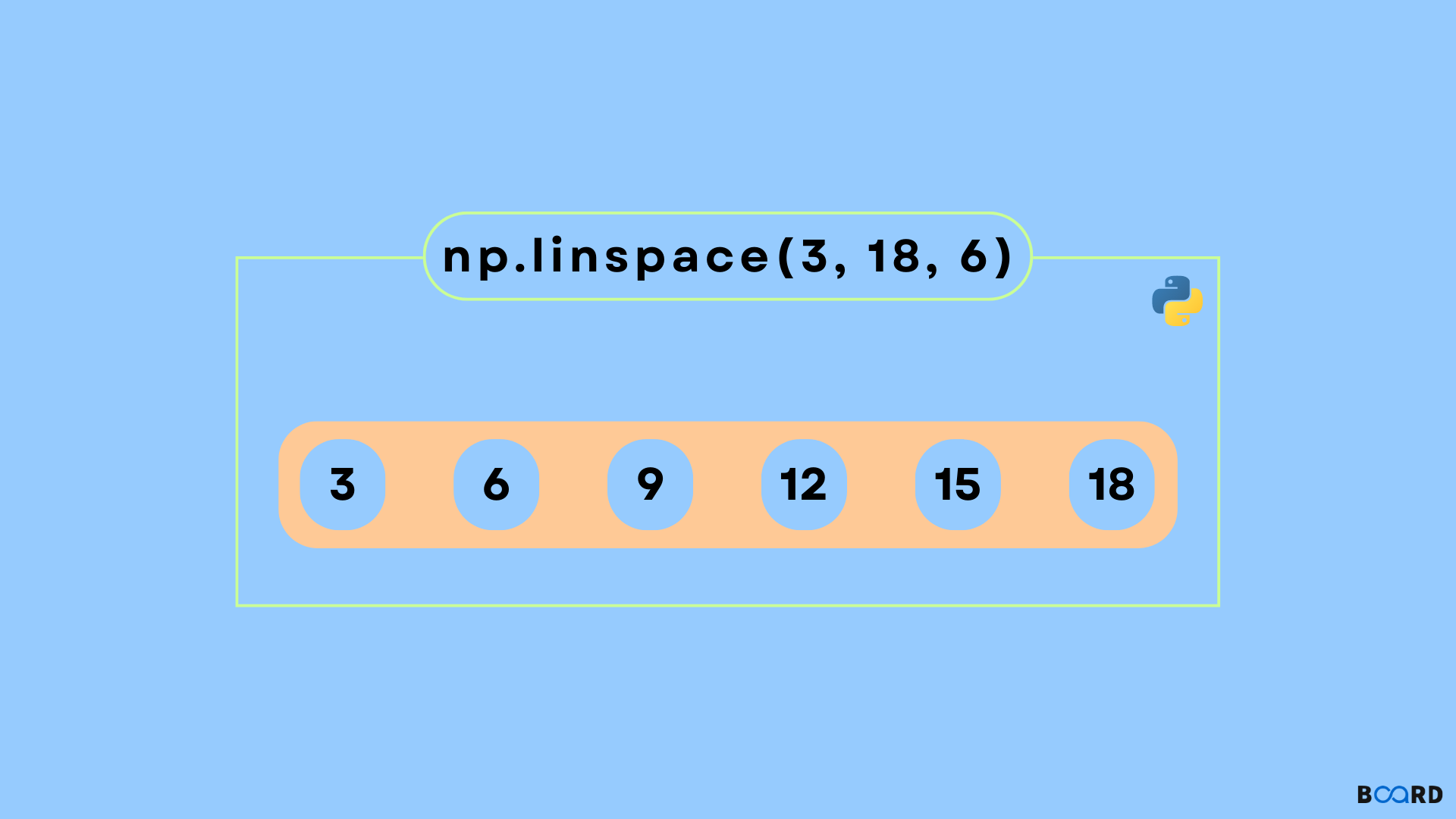# Python numpy.linspace()## Introduction

In this article, we shall learn about the method “linspace” from the module “numpy” in Python. It is a Python method that returns evenly-spaced numbers over a given interval. linspace() is quite similar to the Python arange() function. But a difference in between the two is that linspace() uses a sample number instead of a step.

## Scope

• Syntax of using the linspace() method in Python.
• The parameters that the linspace function takes.
• The return value of the linspace method.
• A fine example of using the linspace() method along with code implementation.

## Syntax

 numpy.linspace(start, stop, num, endpoint, retstep, dtype)

## Parameters

start

It is an optional parameter and denotes the start of the range of the interval. Its default value is zero.

stop

It represents the end of the interval range.

restep

If set to True, returns (samples, step). The default value is False.

num

It’s an optional parameter the value of which must be a number dictating the number of samples to be generated.

dtype

It’s the type of output array.

## Return

 ndarraystep: If restep were set to True, [float, optional]

## Implementation and examples

### Example 1

 import numpy as npprint(np.linspace(2.0, 3.0, num=5, retstep=True))item =np.linspace(0, 2, 10)print(np.sin(item))

Output

 (array([2.  , 2.25, 2.5 , 2.75, 3.  ]), 0.25)[0.         0.22039774 0.42995636 0.6183698  0.77637192 0.8961922 0.9719379  0.99988386 0.9786557  0.9092974

### Example 2

 import numpy as npimport pylab as plx = np.linspace(0, 2, 10, endpoint = False)y = np.ones(10)pl.plot(x, y, '*')pl.xlim(-0.2, 1.8)